# GENERATION-RECOMBINATION NOISE AND OTHER FEATURES OF DOPED SILICON IN A WIDE TEMPERATURE RANGE

V. Palenskis, J. Glemža, and J. Matukas

Institute of Applied Electrodynamics and Telecommunications, Vilnius University, Saulėtekio 3, 10257 Vilnius, Lithuania
Email: jonas.matukas@ff.vu.lt; vilius.palenskis@ff.vu.lt; justinas.glemza@ff.vu.lt

Received 6 July 2022; revised 12 September 2022; accepted 12 September 2022

The characteristics of the generation-recombination (g-r) process in silicon are investigated in a temperature range from 25 to 360 K. In the case of shallow donors, it is shown that the free electron density strongly depends on temperature: only 20% of donors are ionized at shallow donor densities of about 1017 cm–3 at liquid nitrogen temperature. The maximum of the variance of generation-recombination noise due to the free electron density fluctuations for a silicon sample with shallow donors strongly increases with donor density and shifts with temperature. It is demonstrated that the relative variance of free electron number fluctuations is always equal to 0.5 at low temperatures. The normalized generation-recombination noise spectra are depicted in a very wide frequency range. There is also a detailed investigation of the generation-recombination noise characteristics of an acceptor-partially compensated silicon sample with two donor levels. In this work, the main focus is on the characteristics of silicon doped by shallow donors as it is extremely widely used.

Keywords: free electron density fluctuations, generation-recombination noise, shallow donors, silicon

PACS: 72.70.+m, 72.20.Jv

## 1. Introduction

Although the processes of free charge carrier generation (emission) and recombination (capture) have been investigated over the last seventy years , many experiments have been performed with various semiconductor materials  and devices , and explanations are provided. During the  generation-recombination (g-r) process, the free charge carrier number fluctuations keep the  charge neutrality in the  total sample. This term is usually used for describing the free charge carrier number fluctuations caused by donor or acceptor levels. The  free charge carrier number fluctuations in equilibrium also produce fluctuations in the resistance of the sample. These resistance fluctuations can be simply measured by using direct current because they produce the  flowing current fluctuations which are proportional to the  square of the  magnitude of the direct current. In the case of the deep defect levels, the  generation-recombination process is usually described as a charge carrier emission and capture, or retrapping process. In the latter case, the free charge carrier fluctuations are caused by localized defect states with different energy levels in the band gap and are characterized by various free carrier relaxation times . The free charge carrier emission-capture process is also thermally activated and does not change the neutrality condition in the sample. According to Refs. , the relaxation times of the g-r process for silicon monocrystals are distributed in the time interval from 0.1 s to 10 μs. In Ref. , it is demonstrated that the charge carrier capture-emission process is the main source of the low-frequency noise in homogeneous semiconductors and this process can produce the free charge carrier mobility fluctuations in particular cases .

In this work, important properties of the generation-recombination process in a  silicon sample doped with shallow donors will be presented. The main focus here will be on the temperature dependence of the generation-recombination noise.

## 2. Temperature dependence of the generation-recombination noise

### 2.1. Silicon sample with one partially ionized donor level

Due to the effect of the thermal lattice vibration in donor-type semiconductors some electrons are excited from donor energy levels into the conduction band (generation process), and at the  same time there happens the reverse process (recombination): some electrons are captured by ionized donors. To estimate the characteristics of the g-r noise, it is sufficient to find the  generation g(n) and recombination r(n) rate expressions from the physical model.

Consider the  g-r noise temperature properties for a  donor-type silicon sample with the  volume = 0.01 cm3 and the donor density nd = 1015 cm–3 at the energy level Ed = 0.1 eV below the conduction band (Fig. 1). In the general case, the average free electron density n0 is described by the following equation :

where εd = Ed/kT, η = EF/kT (here EF is the Fermi level energy), and n0d is the neutral donor density, for donors β–1 = 2.

${N}_{\text{c}}=2{\left(2\pi mkT/{h}^{2}\right)}^{3/2},\text{\hspace{0.17em}}\text{\hspace{0.17em}}\text{\hspace{0.17em}}\text{\hspace{0.17em}}\text{\hspace{0.17em}}\text{\hspace{0.17em}}\text{\hspace{0.17em}}\text{\hspace{0.17em}}\text{\hspace{0.17em}}\text{\hspace{0.17em}}\text{\hspace{0.17em}}\left(2\right)$

For silicon

Dependences of the free electron density n0 and the Fermi energy EF on temperature for silicon with nd = 1015 cm–3 at the energy level Ed = 0.1 eV are presented in Fig. 2. When the Fermi energy level coincides with the donor energy level, the free electron density in the conduction band is n0 = nd/3. As Fig. 2 shows, all donors are ionized at temperature > 200 K. In the very low temperature range, Eq. (1) can be approximated as

${n}_{0}\approx {\left(\beta {n}_{\text{d}}{N}_{\text{c}}\right)}^{1/2}\text{exp}\left(-{E}_{\text{d}}/2kT\right).\text{\hspace{0.17em}}\text{\hspace{0.17em}}\text{\hspace{0.17em}}\text{\hspace{0.17em}}\text{\hspace{0.17em}}\text{\hspace{0.17em}}\text{\hspace{0.17em}}\text{\hspace{0.17em}}\text{\hspace{0.17em}}\text{\hspace{0.17em}}\text{\hspace{0.17em}}\text{\hspace{0.17em}}\text{\hspace{0.17em}}\text{\hspace{0.17em}}\text{\hspace{0.17em}}\text{\hspace{0.17em}}\text{\hspace{0.17em}}\text{\hspace{0.17em}}\text{\hspace{0.17em}}\text{\hspace{0.17em}}\text{\hspace{0.17em}}\text{\hspace{0.17em}}\text{\hspace{0.17em}}\text{\hspace{0.17em}}\left(4\right)$

The rate of free electron generation g(n) is proportional to the non-ionized donor density nd – nd+, i.e. to the density of electrons in the donor energy level Ed:

$g\left(n\right)=a\left({n}_{\text{d}}-\mid {n}_{\text{d}}^{+}\right)=a\left({n}_{\text{d}}-n\right).\text{\hspace{0.17em}}\text{\hspace{0.17em}}\text{\hspace{0.17em}}\text{\hspace{0.17em}}\text{\hspace{0.17em}}\text{\hspace{0.17em}}\text{\hspace{0.17em}}\text{\hspace{0.17em}}\text{\hspace{0.17em}}\text{\hspace{0.17em}}\text{\hspace{0.17em}}\text{\hspace{0.17em}}\text{\hspace{0.17em}}\text{\hspace{0.17em}}\text{\hspace{0.17em}}\text{\hspace{0.17em}}\text{\hspace{0.17em}}\text{\hspace{0.17em}}\text{\hspace{0.17em}}\text{\hspace{0.17em}}\text{\hspace{0.17em}}\text{\hspace{0.17em}}\text{\hspace{0.17em}}\text{\hspace{0.17em}}\text{\hspace{0.17em}}\text{\hspace{0.17em}}\text{\hspace{0.17em}}\text{\hspace{0.17em}}\text{\hspace{0.17em}}\text{\hspace{0.17em}}\text{\hspace{0.17em}}\text{\hspace{0.17em}}\left(5\right)$

Here a is the  proportionality coefficient, and n0 + ∆n is a fluctuating quantity. And the rate of electron recombination r(n) is proportional to the density of the free electrons and to the empty (ionized) donor centre density ${n}_{\text{d}}^{+}$  = n:

$r\left(n\right)=\gamma n\cdot {n}_{\text{d}}^{+}=\gamma {n}^{2}.\text{\hspace{0.17em}}\text{\hspace{0.17em}}\text{\hspace{0.17em}}\text{\hspace{0.17em}}\text{\hspace{0.17em}}\text{\hspace{0.17em}}\text{\hspace{0.17em}}\text{\hspace{0.17em}}\text{\hspace{0.17em}}\text{\hspace{0.17em}}\text{\hspace{0.17em}}\text{\hspace{0.17em}}\text{\hspace{0.17em}}\text{\hspace{0.17em}}\text{\hspace{0.17em}}\text{\hspace{0.17em}}\text{\hspace{0.17em}}\left(6\right)$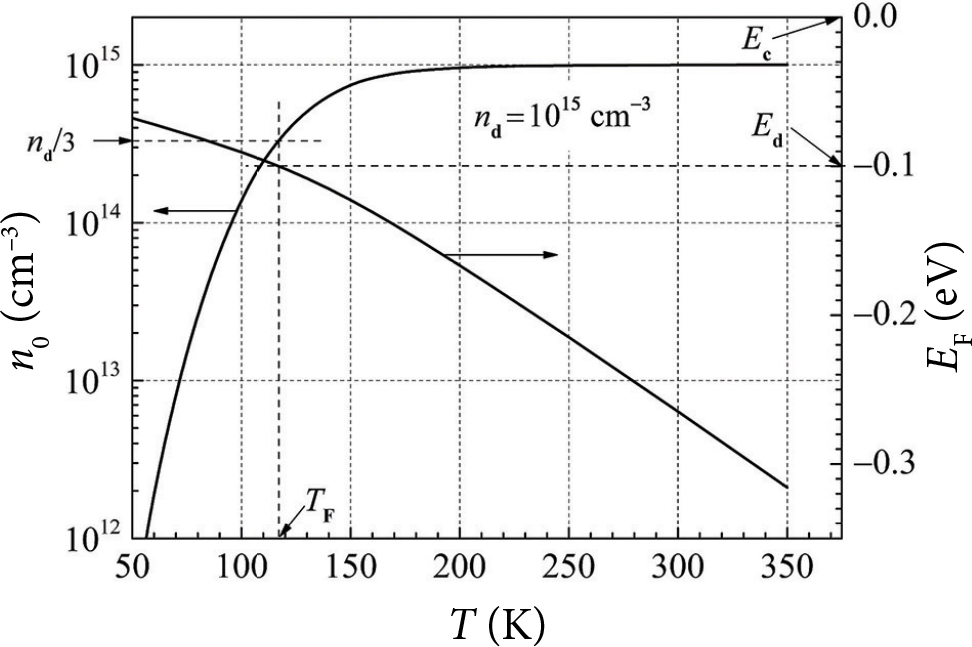Fig. 2. Dependences of the free electron density n0 (left scale) and the Fermi energy EF (right scale) on temperature for silicon with the donor density nd = 1015 cm–3 at the energy level Ed = 0.1 eV (here TF is the temperature when the Fermi level energy coincides with the donor level energy).

Here γ is the recombination coefficient: γ = vTσs, vT is the electron thermal velocity, and σs is the electron capture cross-section. The coefficient a can be found from the equilibrium condition g(n0) = r(n0):

$a=\gamma \frac{{n}_{0}{}^{2}}{{n}_{d}-{n}_{0}}.\text{\hspace{0.17em}}\text{\hspace{0.17em}}\text{\hspace{0.17em}}\text{\hspace{0.17em}}\text{\hspace{0.17em}}\text{\hspace{0.17em}}\text{\hspace{0.17em}}\text{\hspace{0.17em}}\text{\hspace{0.17em}}\text{\hspace{0.17em}}\text{\hspace{0.17em}}\text{\hspace{0.17em}}\text{\hspace{0.17em}}\text{\hspace{0.17em}}\left(7\right)$

The relaxation time of free electrons is estimated in the following way ,

$\begin{array}{l}{\tau }_{0}=\frac{1}{r\text{'}\left({n}_{0}\right)-g\text{'}\left({n}_{0}\right)}=\frac{1}{2\gamma {n}_{0}+a}\hfill \\ =\frac{{n}_{d}-{n}_{0}}{\gamma {n}_{0}\left(2{n}_{d}-{n}_{0}\right)},\hfill \end{array}\text{\hspace{0.17em}}\text{\hspace{0.17em}}\text{\hspace{0.17em}}\text{\hspace{0.17em}}\text{\hspace{0.17em}}\text{\hspace{0.17em}}\text{\hspace{0.17em}}\text{\hspace{0.17em}}\text{\hspace{0.17em}}\text{\hspace{0.17em}}\text{\hspace{0.17em}}\text{\hspace{0.17em}}\text{\hspace{0.17em}}\text{\hspace{0.17em}}\text{\hspace{0.17em}}\text{\hspace{0.17em}}\text{\hspace{0.17em}}\text{\hspace{0.17em}}\text{\hspace{0.17em}}\text{\hspace{0.17em}}\text{\hspace{0.17em}}\text{\hspace{0.17em}}\text{\hspace{0.17em}}\text{\hspace{0.17em}}\text{\hspace{0.17em}}\text{\hspace{0.17em}}\left(8\right)$

and the variance of the free electron density fluctuations is calculated as

$〈\Delta {n}^{2}〉=g\left({n}_{0}\right){\tau }_{0}/V=\frac{{n}_{0}\left({n}_{d}-{n}_{0}\right)}{\left(2{n}_{d}-{n}_{0}\right)V},\text{\hspace{0.17em}}\text{\hspace{0.17em}}\text{\hspace{0.17em}}\text{\hspace{0.17em}}\text{\hspace{0.17em}}\text{\hspace{0.17em}}\text{\hspace{0.17em}}\text{\hspace{0.17em}}\text{\hspace{0.17em}}\text{\hspace{0.17em}}\text{\hspace{0.17em}}\text{\hspace{0.17em}}\text{\hspace{0.17em}}\text{\hspace{0.17em}}\text{\hspace{0.17em}}\text{\hspace{0.17em}}\text{\hspace{0.17em}}\text{\hspace{0.17em}}\text{\hspace{0.17em}}\text{\hspace{0.17em}}\text{\hspace{0.17em}}\text{\hspace{0.17em}}\text{\hspace{0.17em}}\left(9\right)$

where = 0.01 cm3. Including the fact that relaxation time is approximately inversely proportional to n0, and γ ~ T1/2, dependences of the relaxation time τ0 and τ0 multiplied by a factor (T/295)5/4 on reciprocal temperature (1000/T) are depicted in Fig. 3. When 1000/T > 7 (or T < 40 K), the relaxation time exponentially increases with temperature.

Temperature dependences of the  variance of free electron density fluctuations and the  Fermi energy for silicon with the donor impurity density 1015 cm−3 at Ed = 0.1 eV are represented in Fig. 4(a). The maximum of the free carrier fluctuation variance is observed at the  temperature T1  =  136  K when EF/Ed  =  E1/Ed  ≈  1.1. However, EF  =  Ed at the temperature TF = 116.5 K, i.e. the maximum of the fluctuation variance appears when the Fermi level energy is below the donor level energy.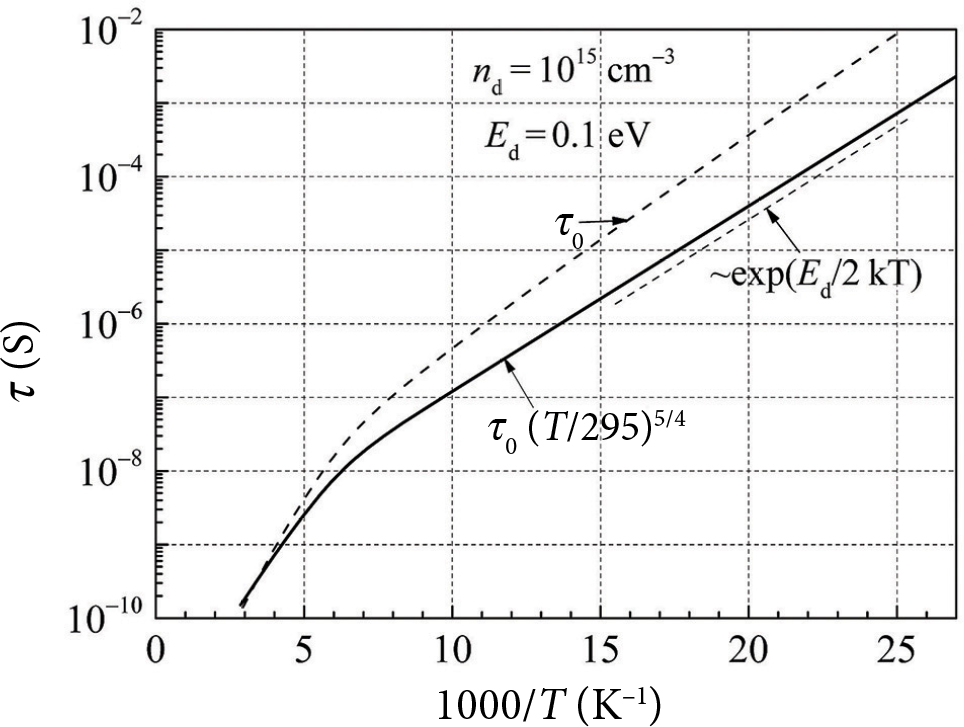Fig. 3. Dependences of the free electron relaxation time τ0 and the normalized relaxation time τ0(T/295)5/4 on reciprocal temperature (1000/T) for silicon with the donor density nd = 1015 cm−3 at the energy level Ed = 0.1 eV.

From Eq. (9) it follows that the relative variance of free electron number N fluctuations in the silicon sample with the number of donors Nd can be expressed as

$\frac{〈\text{Δ}{N}^{2}〉}{{N}_{0}}=\frac{{N}_{d}-{N}_{0}}{2{N}_{d}-{N}_{0}}.\text{\hspace{0.17em}}\text{\hspace{0.17em}}\text{\hspace{0.17em}}\text{\hspace{0.17em}}\text{\hspace{0.17em}}\text{\hspace{0.17em}}\text{\hspace{0.17em}}\text{\hspace{0.17em}}\text{\hspace{0.17em}}\text{\hspace{0.17em}}\text{\hspace{0.17em}}\text{\hspace{0.17em}}\text{\hspace{0.17em}}\text{\hspace{0.17em}}\text{\hspace{0.17em}}\text{\hspace{0.17em}}\text{\hspace{0.17em}}\text{\hspace{0.17em}}\text{\hspace{0.17em}}\text{\hspace{0.17em}}\text{\hspace{0.17em}}\text{\hspace{0.17em}}\left(10\right)$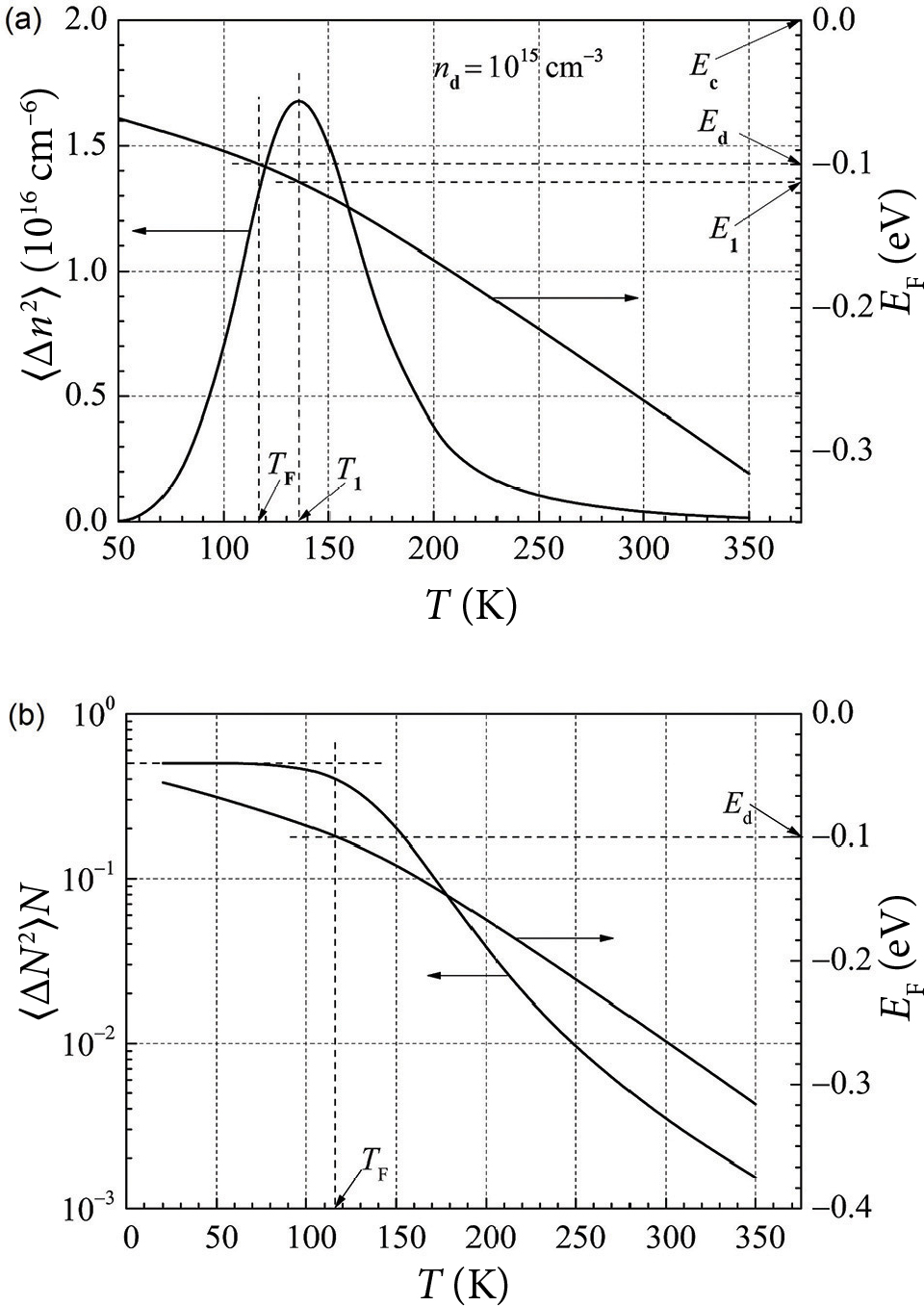Fig. 4. Temperature dependences of (a) the  variance of free electron density fluctuations 〈∆n2〉 and the  Fermi energy EF, and (b) the  relative variance of free electron number fluctuations 〈∆N2〉/N and the Fermi energy EF for silicon with the donor density 1015 cm−3 at the energy level Ed = 0.1 eV (there TF is the  temperature when the  Fermi level energy coincides with the donor level energy, and T1 is the temperature when the  variance of free electron density fluctuations reaches the maximum value).

This relation 〈∆N2〉/N0 = 0.5 when N0Nd. The dependence of the relative variance of free electron number fluctuations on temperature is shown in Fig.  4(b), and it clearly illustrates that 〈∆N2〉/N0 = 0.5 when the Fermi level energy is over the donor level energy. For random particles with Pois-son’s law of distribution, this ratio is equal to one. The  smaller ratio for the  electrons in the  donor silicon is due to the degeneracy factor β = 1/2. For a silicon sample with one donor level Ed = 0.1 eV at temperature < 120 K, the Fermi level energy is always over the donor level energy (Fig. 2). There the  free electron density exponentially decreases with temperature decreasing (Fig. 2), while the relaxation time exponentially increases (Fig. 3). In this temperature range, the relative variance of free electron number fluctuations 〈ΔN2〉/N0 = 0.5. The maximum of the variance of free electron density fluctuations 〈Δn2〉 occurs when the Fermi level energy is about 10% below the donor level energy (Fig. 4(a)).

For the g-r noise spectrum calculation, it is convenient to present the results as a normalized power spectral density (multiplied by frequency):

$\frac{{S}_{N}\left(f\right)}{{N}_{0}^{2}}×f=4\frac{〈\text{Δ}{n}^{2}〉}{{n}_{0}^{2}}\cdot \frac{f{\tau }_{0}}{1+{\left(\omega {\tau }_{0}\right)}^{2}}.\text{\hspace{0.17em}}\text{\hspace{0.17em}}\text{\hspace{0.17em}}\text{\hspace{0.17em}}\text{\hspace{0.17em}}\text{\hspace{0.17em}}\text{\hspace{0.17em}}\text{\hspace{0.17em}}\text{\hspace{0.17em}}\text{\hspace{0.17em}}\text{\hspace{0.17em}}\text{\hspace{0.17em}}\text{\hspace{0.17em}}\text{\hspace{0.17em}}\text{\hspace{0.17em}}\text{\hspace{0.17em}}\text{\hspace{0.17em}}\text{\hspace{0.17em}}\text{\hspace{0.17em}}\text{\hspace{0.17em}}\text{\hspace{0.17em}}\text{\hspace{0.17em}}\text{\hspace{0.17em}}\text{\hspace{0.17em}}\text{\hspace{0.17em}}\text{\hspace{0.17em}}\text{\hspace{0.17em}}\left(11\right)$

The frequency dependences of the normalized power spectral density of free electron number fluctuations for a silicon sample at different temperatures are presented in Fig. 5.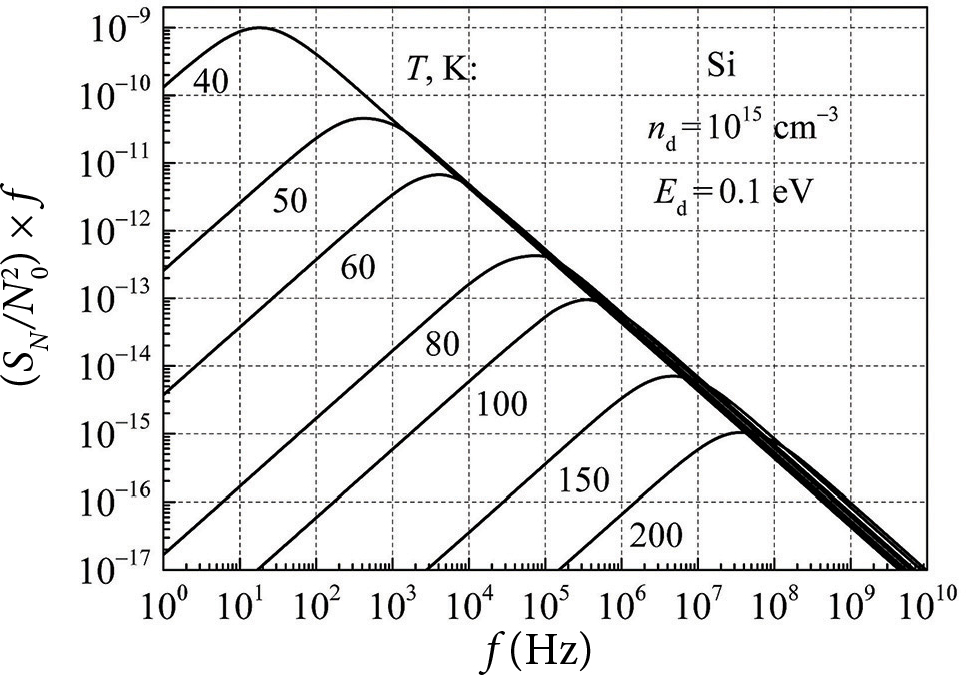Fig. 5. Normalized spectra of free electron number fluctuations for a silicon sample with the donor density nd = 1015 cm−3 at the energy level Ed = 0.1 eV at different temperatures.

The maximum of the normalized spectral density of free electron number fluctuations decreases and shifts to higher frequencies with increasing temperature (Fig. 5). The peak values are observed at frequencies f0 = 1/(2πτ0). By presenting the measurement results in this way, it is very easy to determine the relaxation time τ0.

### 2.2. G-r properties in silicon with various shallow donor densities

At high shallow donor densities, donor ions interact with each other, causing their wave functions to overlap and the donor energy level to split . This results in a decreased ionization energy and the formation of the band tail at the bottom of the conduction band rather than a well-defined band edge. Taking into account that the donor atoms replace the silicon atoms in the lattice , the donor levels cannot be considered as additional levels to the levels of the conduction band. These donor levels partly overlap with the energy levels in the conduction band, and when Ed = 0, the donor energy states completely overlap the conduction band, and all valence electrons are in the conduction band. The decrease of the shallow donor level energy (Ed0 = 0.05 eV) with the increase in donor density for the silicon sample is presented in Fig. 6(a) and can be described as 

${E}_{d}=0.05-2.33\cdot {10}^{-8}{n}_{d}{}^{1/3},\text{eV}.\text{\hspace{0.17em}}\text{\hspace{0.17em}}\text{\hspace{0.17em}}\text{\hspace{0.17em}}\text{\hspace{0.17em}}\text{\hspace{0.17em}}\text{\hspace{0.17em}}\text{\hspace{0.17em}}\text{\hspace{0.17em}}\text{\hspace{0.17em}}\text{\hspace{0.17em}}\text{\hspace{0.17em}}\text{\hspace{0.17em}}\text{\hspace{0.17em}}\text{\hspace{0.17em}}\text{\hspace{0.17em}}\left(12\right)$

Temperature dependences of the  free electron density and the Fermi level energy at three different shallow donor densities, 1013, 1015 and 1017  cm−3, in silicon are presented in Fig.  6(b). The dots A1, A2 and A3 represent the free electron densities at temperatures TF1, TF2 and TF3, respectively, when the Fermi level energy coincides with the donor level energy and Eq. (12) is taken into account. Similarly, the dots B1, B2 and B3 denote the Fermi level energies when they coincide with the donor level energies and Eq. (12) is also taken into account. Therefore, it is clear that the relation n0 = (1/3) nd is perfectly fulfilled for various presented donor densities when the Fermi level energy coincides with the donor level energy.

Temperature dependences of the relative free electron density n0/nd for three different donor densities are shown in Fig.  7(a). Usually, it is considered that all donors with a shallow energy level of 0.05  eV are completely ionized at temperatures above liquid nitrogen. As Fig. 7(a) indicates, the requirement for 95% of donors to be ionized at temperatures above liquid nitrogen is only fulfilled at low (≤1013 cm–3) donor densities. This ionization level for donor density of 1015 cm–3 is only achieved at temperatures above 114 K, and for 1017 cm–3 only at temperatures above 252 K. Thus, the  statement that all shallow donors are ionized at temperatures above liquid nitrogen should be used with extreme caution. What determines that not all donors are ionized at higher shallow donor density? The  higher the  shallow donor density, the  lower the  relative free electron density (Fig. 7)(a): only 20% of donors are ionized at the  temperature of liquid nitrogen when donor density is 1017  cm–3. The  dependence of the  conduction band states filling func tion g(Ef (E) on energy at T = 100 K is presented in Fig. 7(b). At low donor densities, electrons fill energy states near the bottom of the conduction band. However, for higher donor densities, some electrons must be excited to the higher energy levels of the conduction band because the energy levels near the bottom of the conduction band are occupied.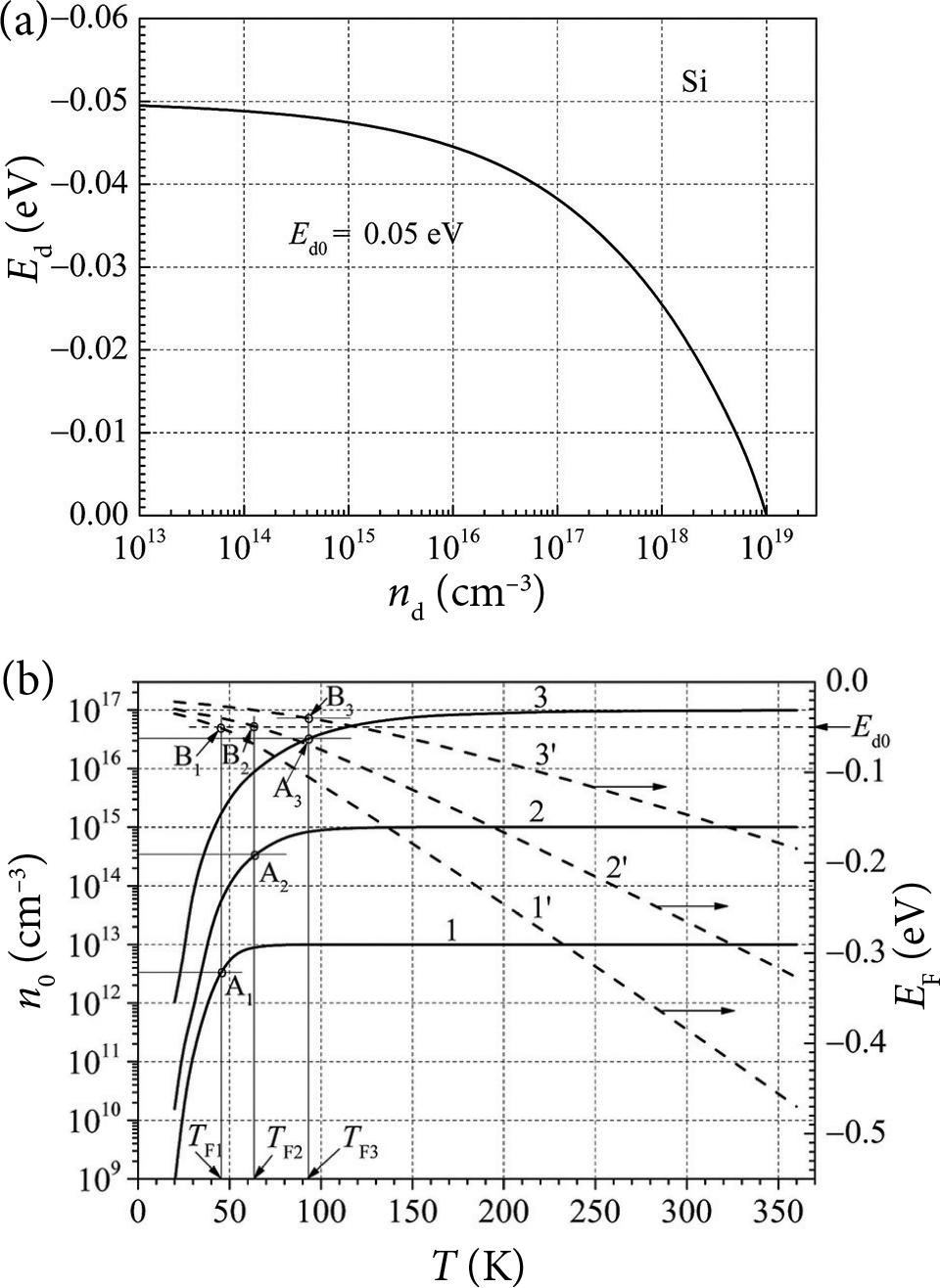Fig. 6. (a) The decrease of the shallow donor level energy Ed with the increase in donor density at room temperature; (b) temperature dependences of the free electron density (left scale) and the Fermi level energy (right scale) at three different donor densities, in cm–3: 1 at 1013; 2 at 1015; 3 at 1017 (the donor energy decrease is taken into account).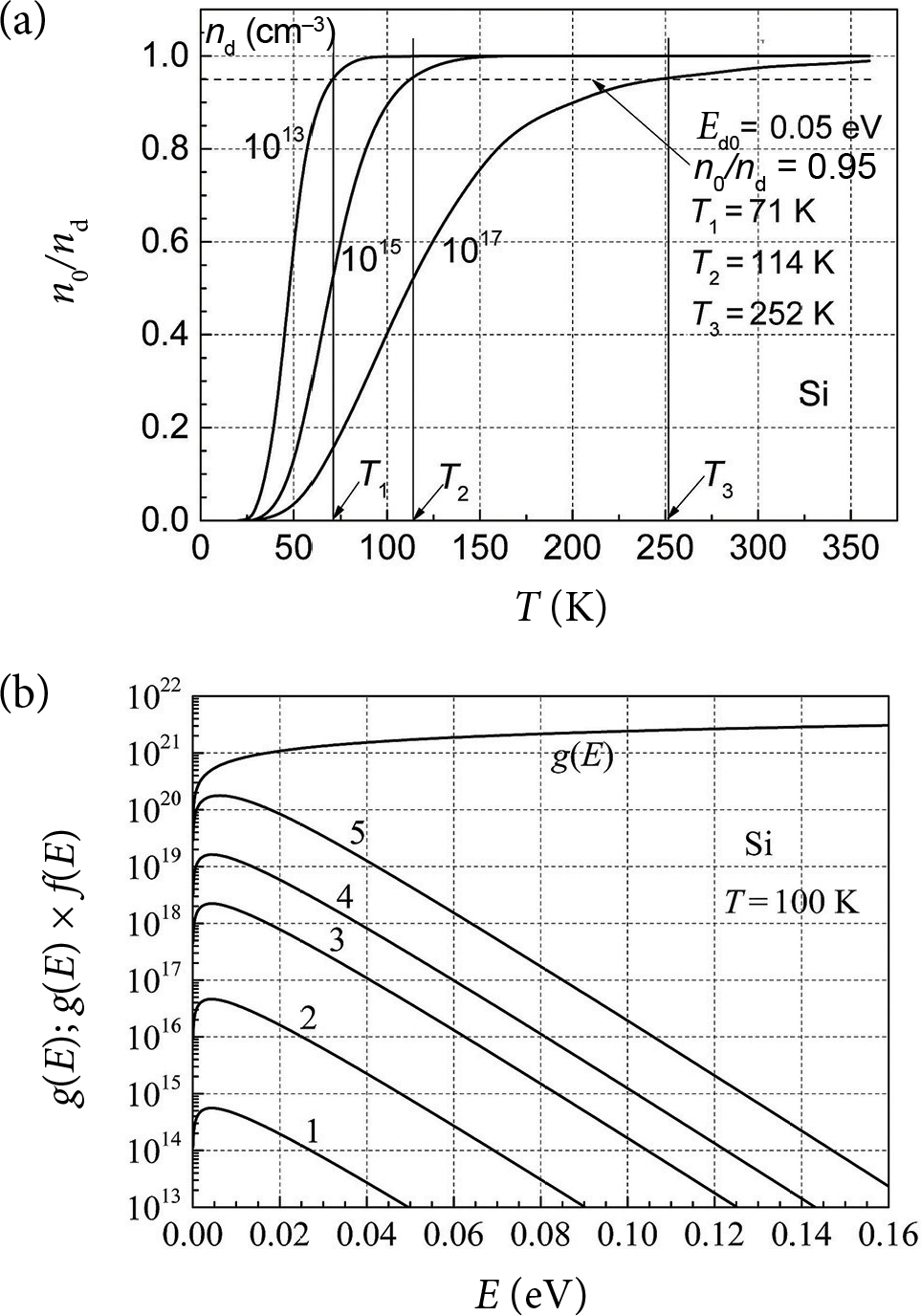Fig. 7. (a) Temperature dependences of the  relative density of the free electrons n0/nd for three different donor densities; (b) dependences of the  density of states g(E) and the states filling function g(E)f(E) on energy at T = 100 K in silicon for various donor densities, cm–3: 1 for 1013 (–0.114 eV); 2 for 1015 (–0.075 eV); 3  for  1017 (–0.0423  eV); 4  for  1018 (–0.0252  eV); 5 for 1019 (–0.00131 eV), here in brackets the donor energy values taking into account the donor level energy decrease by Eq. (12) are presented.

Temperature dependence of the  free electron density for high shallow donor densities in silicon, which is estimated by Eq. (1), is depicted in Fig. 8(a). The Hall effect measurement data show that all donors are ionized when donor density is 1019 cm–3 . Thus, Eq. (1) incorrectly describes the free electron density at high donor densities in silicon. Then, the expression for the free electron density can be estimated as

where the probability multiplier [1 – exp(–εd)] shows that at Ed = 0, i.e. the donor energy states overlap with the conduction band levels. Considering that every donor changes the silicon atom (substitutional impurity)  and that donor levels are formed as decoupled levels from the conduction band, the total density of states due to doping changes weakly . It is confirmed by electronic heat measurements of silicon with a high donor doping .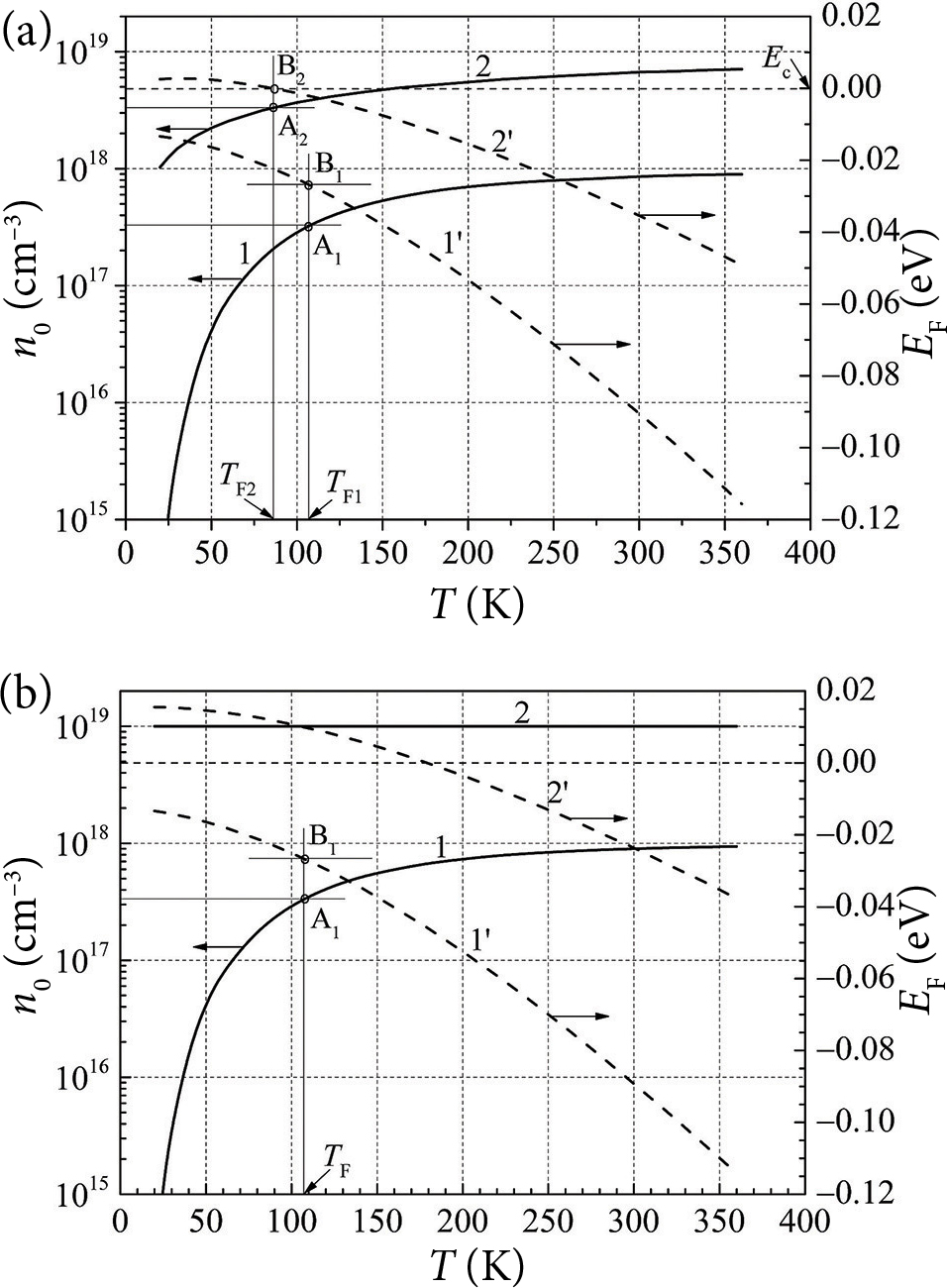Fig. 8. (a) Temperature dependences of the free electron density (left scale) and the  Fermi level energy (right scale) for high densities of shallow donors, in cm–3: 1 for 1018 (Ed = 0.025 eV); 2 for 1019 (Ed = 0) calculated by Eq. (1); (b) the same as in Fig. 8(a), but calculated by Eq. (13). Dots A1 and A2 on curves n0(T) show the free electron densities when the Fermi level energy coincides with the donor level energy (denoted by dots B1 and B2).

The temperature dependence of the  free electron density for high donor densities estimated by Eq. (13) is presented in Fig. 8(b) and it is in good agreement with the experimental data . Equation (13) is valid for any donor density with a low or high donor level energy. Equations (1) and (13) completely coincide at shallow donor densities ≤1017 cm–3. To evaluate the density of the free electrons for any donor density, it is convenient to have an analogous expression for the function F1/2(η),

where the parameter

Dependences of the functions exp(η), F1/2(η) and α on η are presented in Fig. 9(a). The difference between the  approximation curve (Eq.  (15)) and the  ratio exp(η)/F1/2(η) does not exceed 5% in the whole η range (η ≤ 15). At η values below –3, the ratio exp(η)/F1/2(η) = 1. Temperature depend-ences of the  free electron density calculated by Eq. (13) for five different donor densities are presented in Fig. 9(b). The obtained data are in good agreement with the experimental Hall effect measurement results . The Fermi level energy for all donor densities has negative values, but for nd = 1019 cm–3 it becomes positive at temperatures below 150 K.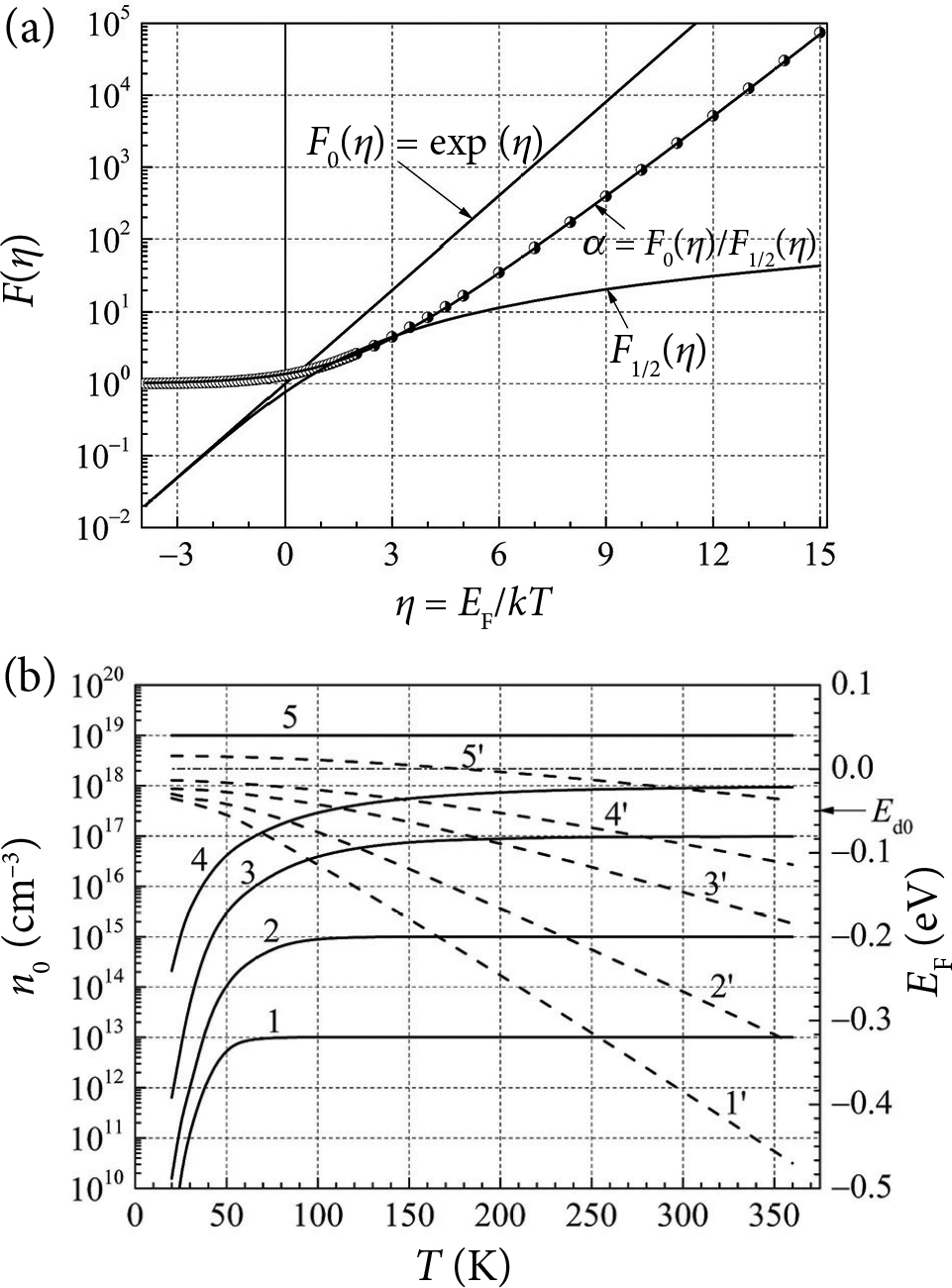Fig. 9. (a) Dependences of functions expη, F1/2(η) and α on η (dots on α curve denote the calculated ratio expη/ F1/2(η), solid α line is the approximation by Eq. (15)); (b) temperature dependences of the free electron density (calculated by Eq. (13), left scale) and the Fermi level energy (right scale) for various shallow donor densities nd, in cm–3: 1 for 1013; 2 for 1015; 3 for 1017; 4 for 1018; 5 for 1019 (here Eq. (12) has also been taken into account).

Temperature dependences of the  variance of electron density fluctuations and the relative variance of free electron number fluctuations 〈∆N2〉/N0 at three different densities of shallow donors are depicted in Fig. 10. The temperature dependence of the variance of free electron density fluctuations is characterized by a  maximum, which strongly increases (about four orders of magnitude) with increasing donor density and shifts in the temperature range from 50 to 125 K (Fig. 10(a)). The relative variance of free electron number fluctuations 〈∆N2〉/N0 at low temperatures is equal to 0.5, and this value does not depend on the  donor density but decreases strongly at higher temperatures (Fig. 10(b)). It can be pointed out that the value 0.5 reflects a situation where the free electron fluctuations obey Poisson’s law of distribution.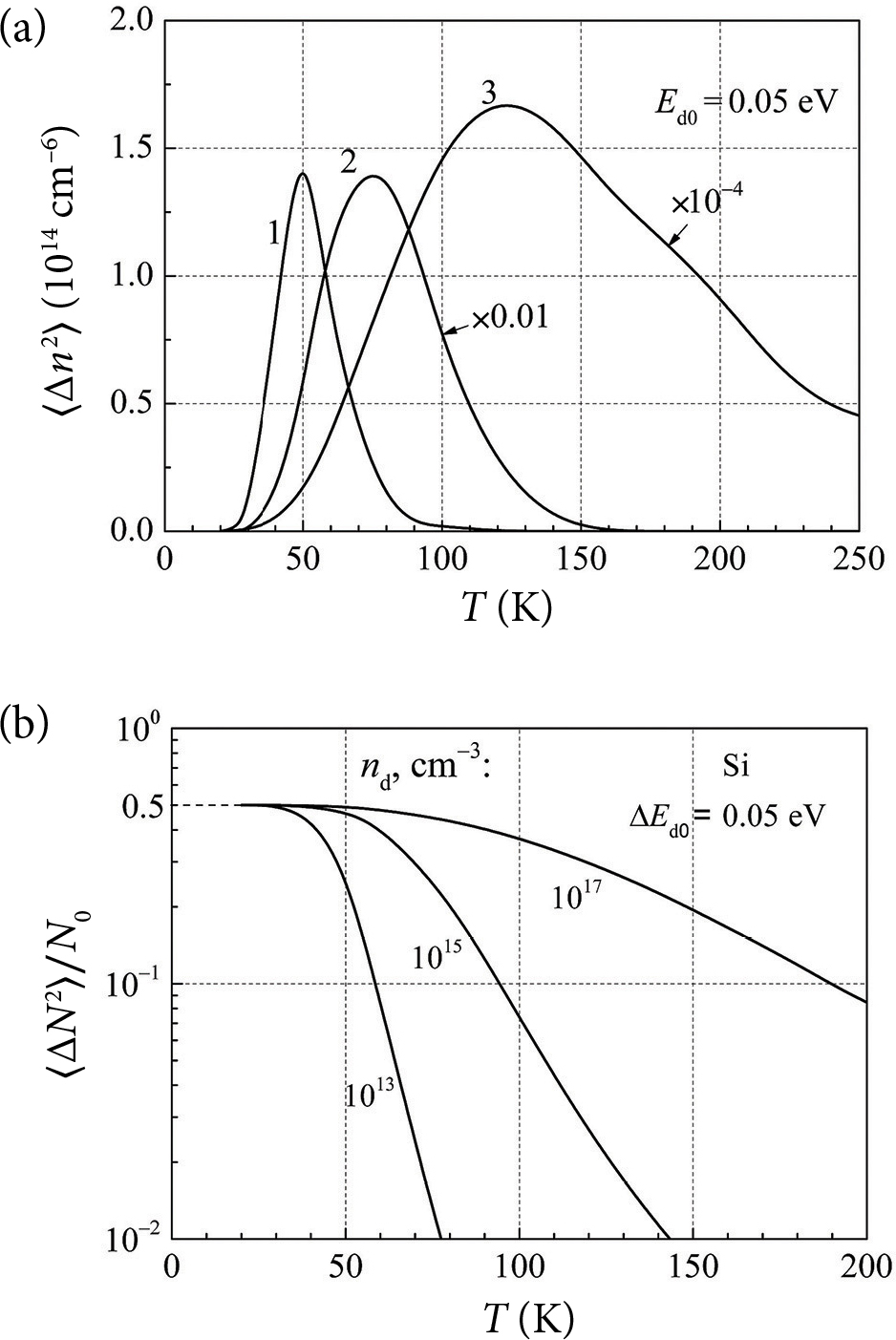Fig. 10. Temperature dependences of (a) the variance of the free electron density 〈Δn2〉 and (b) the relative variance of free electron number fluctuations 〈ΔN2〉/N0 at three different densities of shallow donors (Ed0 = 0.05 eV), in cm–3: 1 at 1013; 2 at 1015; 3 at 1017 (here Eq. (12) has also been taken into account).

### 2.3. Temperature properties of the generation-recombination noise in silicon with two donor levels in the presence of acceptors

A donor doped silicon sample of volume =  0.01  cm3 with the  shallow donor density n1d = 2·1013 cm–3 at the energy level E1d = 0.05 eV and with the deep donor density n2d = 3·1014 cm−3 at the level E2d = 0.25 eV is partially compensated by acceptors with the  density na  =  1.5⋅1013  cm–3 (Fig. 11).

In the general case, the electron generation and recombination from the shallow donor level E1d in the silicon partially compensated by acceptors can be described as

where n01d is additionally decreased due to the capture of electrons by acceptors; the parameter a1 can be found from the equilibrium condition g1(n0) = r1(n0):

Then, the electron relaxation time of the g-r process between the shallow donor level and conduction band can be estimated as

$\begin{array}{l}{\tau }_{1}=\frac{1}{{r}_{1}^{\text{'}}\left(n\right)-{g}_{1}^{\text{'}}\left(n\right)}\hfill \\ =\frac{\left({n}_{1d}-{n}_{a}-{n}_{1d}^{+}\right)/\gamma }{{n}_{1d}\left({n}_{0}+{n}_{1d}^{+}\right)-{n}_{1d}^{+}\left({n}_{1d}^{+}+{n}_{a}\right)},\hfill \end{array}\text{\hspace{0.17em}}\text{\hspace{0.17em}}\text{\hspace{0.17em}}\text{\hspace{0.17em}}\text{\hspace{0.17em}}\text{\hspace{0.17em}}\text{\hspace{0.17em}}\text{\hspace{0.17em}}\text{\hspace{0.17em}}\text{\hspace{0.17em}}\text{\hspace{0.17em}}\text{\hspace{0.17em}}\text{\hspace{0.17em}}\text{\hspace{0.17em}}\text{\hspace{0.17em}}\text{\hspace{0.17em}}\text{\hspace{0.17em}}\text{\hspace{0.17em}}\text{\hspace{0.17em}}\text{\hspace{0.17em}}\text{\hspace{0.17em}}\text{\hspace{0.17em}}\text{\hspace{0.17em}}\text{\hspace{0.17em}}\text{\hspace{0.17em}}\text{\hspace{0.17em}}\text{\hspace{0.17em}}\text{\hspace{0.17em}}\text{\hspace{0.17em}}\text{\hspace{0.17em}}\left(19\right)$

and the free electron variance produced by the shallow donors is

$〈\Delta {n}_{1}^{2}〉={g}_{1}\left({n}_{0}\right){\tau }_{1}/V=\gamma {n}_{0}\left({n}_{1d}^{+}+{n}_{a}\right){\tau }_{1}/V.\text{\hspace{0.17em}}\text{\hspace{0.17em}}\text{\hspace{0.17em}}\text{\hspace{0.17em}}\text{\hspace{0.17em}}\text{\hspace{0.17em}}\text{\hspace{0.17em}}\text{\hspace{0.17em}}\text{\hspace{0.17em}}\text{\hspace{0.17em}}\text{\hspace{0.17em}}\text{\hspace{0.17em}}\text{\hspace{0.17em}}\text{\hspace{0.17em}}\text{\hspace{0.17em}}\text{\hspace{0.17em}}\text{\hspace{0.17em}}\text{\hspace{0.17em}}\left(20\right)$

The electron generation and recombination from the deep donor level E2d can be described in a similar way:

${a}_{\text{2}}=\frac{\gamma {n}_{0}{n}_{2d}^{+}}{{n}_{2d}-{n}_{2d}^{+}},\text{\hspace{0.17em}}\text{\hspace{0.17em}}\text{\hspace{0.17em}}\text{\hspace{0.17em}}\text{\hspace{0.17em}}\text{\hspace{0.17em}}\text{\hspace{0.17em}}\text{\hspace{0.17em}}\text{\hspace{0.17em}}\text{\hspace{0.17em}}\text{\hspace{0.17em}}\text{\hspace{0.17em}}\text{\hspace{0.17em}}\text{\hspace{0.17em}}\text{\hspace{0.17em}}\text{\hspace{0.17em}}\left(23\right)$
$\begin{array}{l}{\tau }_{2}=\frac{1}{{r}_{2}^{\text{'}}\left(n\right)-{g}_{2}^{\text{'}}\left(n\right)}\hfill \\ =\frac{\left({n}_{2d}-{n}_{2d}^{+}\right)/\gamma }{\left({n}_{0}+{n}_{2d}^{+}\right)\left({n}_{2d}-{n}_{2d}^{+}\right)\left({n}_{0}{n}_{2d}^{+}\right)},\hfill \end{array}\text{\hspace{0.17em}}\text{\hspace{0.17em}}\text{\hspace{0.17em}}\text{\hspace{0.17em}}\text{\hspace{0.17em}}\text{\hspace{0.17em}}\text{\hspace{0.17em}}\text{\hspace{0.17em}}\text{\hspace{0.17em}}\text{\hspace{0.17em}}\text{\hspace{0.17em}}\text{\hspace{0.17em}}\text{\hspace{0.17em}}\text{\hspace{0.17em}}\text{\hspace{0.17em}}\text{\hspace{0.17em}}\text{\hspace{0.17em}}\text{\hspace{0.17em}}\text{\hspace{0.17em}}\text{\hspace{0.17em}}\text{\hspace{0.17em}}\text{\hspace{0.17em}}\text{\hspace{0.17em}}\text{\hspace{0.17em}}\text{\hspace{0.17em}}\text{\hspace{0.17em}}\text{\hspace{0.17em}}\text{\hspace{0.17em}}\text{\hspace{0.17em}}\text{\hspace{0.17em}}\text{\hspace{0.17em}}\text{\hspace{0.17em}}\text{\hspace{0.17em}}\text{\hspace{0.17em}}\text{\hspace{0.17em}}\left(24\right)$
$〈\Delta {n}_{2}^{2}〉={g}_{2}\left({n}_{0}\right){\tau }_{2}/V=\gamma {n}_{0}{n}_{2d}^{+}{\tau }_{2}/V.\text{\hspace{0.17em}}\text{\hspace{0.17em}}\text{\hspace{0.17em}}\text{\hspace{0.17em}}\text{\hspace{0.17em}}\text{\hspace{0.17em}}\text{\hspace{0.17em}}\text{\hspace{0.17em}}\text{\hspace{0.17em}}\text{\hspace{0.17em}}\text{\hspace{0.17em}}\text{\hspace{0.17em}}\text{\hspace{0.17em}}\text{\hspace{0.17em}}\text{\hspace{0.17em}}\text{\hspace{0.17em}}\text{\hspace{0.17em}}\text{\hspace{0.17em}}\text{\hspace{0.17em}}\text{\hspace{0.17em}}\text{\hspace{0.17em}}\text{\hspace{0.17em}}\text{\hspace{0.17em}}\text{\hspace{0.17em}}\text{\hspace{0.17em}}\text{\hspace{0.17em}}\text{\hspace{0.17em}}\text{\hspace{0.17em}}\text{\hspace{0.17em}}\left(25\right)$

Considering that the effective density of donors n1d decreases by the value na due to the number of electrons captured by acceptors, the average free electron density can be calculated by the following expression:

$\begin{array}{l}{n}_{0}\left(T\right)=\frac{{n}_{1d}-{n}_{a}}{1+{\beta }^{-1}\text{exp}\left({\epsilon }_{1d}+\eta \right)}\hfill \\ +\frac{{n}_{2d}}{1+{\beta }^{-1}\text{exp}\left({\epsilon }_{2d}+\eta \right)}={N}_{c}{F}_{1/2}\left(\eta \right).\hfill \end{array}\text{\hspace{0.17em}}\text{\hspace{0.17em}}\text{\hspace{0.17em}}\text{\hspace{0.17em}}\text{\hspace{0.17em}}\text{\hspace{0.17em}}\text{\hspace{0.17em}}\text{\hspace{0.17em}}\text{\hspace{0.17em}}\text{\hspace{0.17em}}\text{\hspace{0.17em}}\text{\hspace{0.17em}}\text{\hspace{0.17em}}\text{\hspace{0.17em}}\text{\hspace{0.17em}}\text{\hspace{0.17em}}\text{\hspace{0.17em}}\text{\hspace{0.17em}}\text{\hspace{0.17em}}\text{\hspace{0.17em}}\text{\hspace{0.17em}}\text{\hspace{0.17em}}\text{\hspace{0.17em}}\text{\hspace{0.17em}}\left(26\right)$

Temperature dependences of the average density of free electrons are shown in Fig. 12(a). Dependences of the relaxation times from the donor levels E1d and E2d on the reciprocal temperature (1000/T) are presented in Fig. 12(b). The relaxation time from the shallow donor level exponentially increases when temperature < 50 K (or 1000/> 20). The excitation of free electrons from the deep donor level E2d is noticeable only at > 100 K (or at 1000/< 10). Due to the compensation by acceptors, the free electron density decreases by the amount of the acceptor density (plateau region in Fig. 12(a)). However, as Fig. 12(b) indicates, the relaxation time of free electrons experiences small changes due to the compensation by acceptors.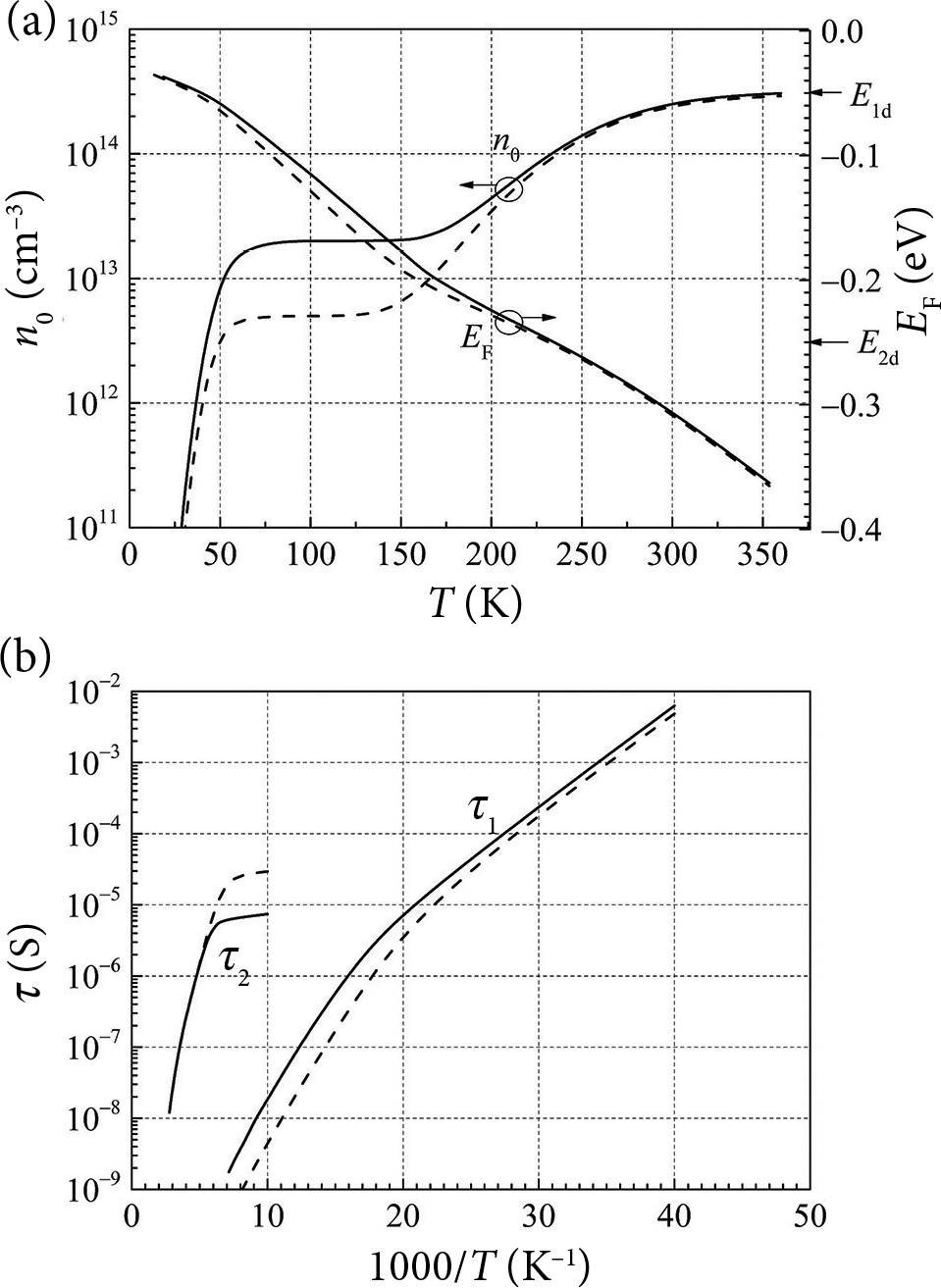Fig. 12. (a) Temperature dependences of the free electron density (left scale) and the Fermi energy (right scale), and (b) dependences of the relaxation times of free electrons from the donor levels on reciprocal temperature (1000/T) in the partially compensated by acceptors na = 1.5·1013 cm–3 (dashed lines) and without the compensation by acceptors (solid lines) silicon sample with the shallow donor density n1d = 2·1013 cm–3 at the energy level E1d = 0.05 eV, and with the deep donor density n2d = 3·1014 cm−3 at the energy level E2d = 0.25 eV.

Temperature dependences of the total variance of free electron density fluctuations 〈∆n2〉 (left scale) and the Fermi level energy (right scale) are represented in Fig. 13(a) (solid line is for the silicon without the  compensation by acceptors; dashed line is for the silicon in the presence of acceptors with density na = 1.5⋅1013 cm–3). The solid line branch of 〈∆n2〉 below = 75 K is caused by the shallow donor level E1d, and when > 75 K, the  branch of 〈∆n2〉 temperature dependence is due to the deep donor level E2d. The minimum in the  temperature dependence of 〈∆n2〉 occurs at ≈  120  K. The  compensation by acceptors significantly shifts the  minimum to ≈  50  K, and the  magnitude of this value decreases by about five orders (Fig. 13(a)). Temperature dependences of the relative variance of the free electron number fluctuations 〈∆N2〉/N0 are shown in Fig. 13(b) (the meaning of solid and dashed lines is the same as in Fig. 13(a)). The solid line branch of 〈∆N2〉/N0 at T < 120 K is caused by the fluctuations of the free electron number from the shallow donor level, and the branch of 〈∆N2〉/N0 at T > 120 K is caused by the fluctuations of the free electron number from the  deep donor level. The  temperature dependence of 〈∆N2〉/N0 has a minimum at ≈ 125 K for the silicon without acceptors. The compensation by acceptors also noticeably shifts this minimum to the lower temperature ≈ 65 K, and the magnitude of the minimum value decreases by about five orders. When the Fermi level energy exceeds E1d, then 〈∆N2〉/N0 ≈ 0.5 for the non-compensated silicon as in the  case of silicon with one donor level.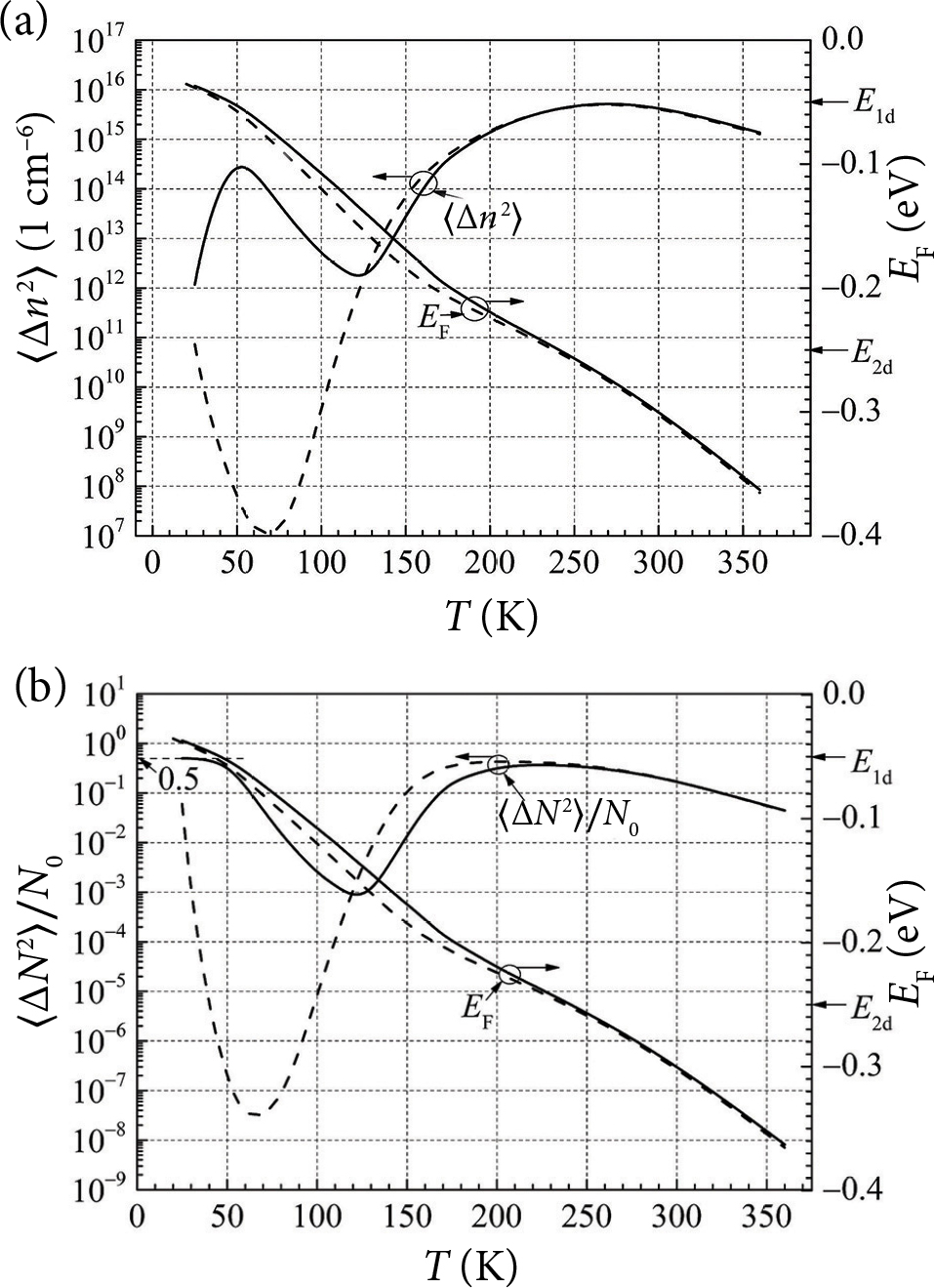Fig. 13. Temperature dependences of (a) the  total variance of free electron density fluctuations 〈∆n2〉, and (b) the relative variance of free electron number fluctuations 〈∆N2〉/N0 in the  partially compensated by acceptors na = 1.5⋅1013 cm–3 (dashed lines) and in the  acceptors free (solid lines) silicon sample with the shallow donor density n1d = 2·1013 cm–3 at the energy level E1d = 0.05 eV, and with the deep donor density n2d = 3·1014 cm−3 at the energy level E2d = 0.25 eV.

The normalized spectral density of free electron number fluctuations in this case can be described as

$\begin{array}{l}\frac{{S}_{N}\left(f\right)}{{N}_{0}^{2}}×f=4\frac{〈\Delta {n}_{1}^{2}〉}{{n}_{0}^{2}}\cdot \frac{f{\tau }_{1}}{1+{\left(\omega {\tau }_{1}\right)}^{2}}\hfill \\ +4\frac{〈\Delta {n}_{2}^{2}〉}{{n}_{0}^{2}}\cdot \frac{f{\tau }_{2}}{1+{\left(\omega {\tau }_{2}\right)}^{2}}.\hfill \end{array}\text{\hspace{0.17em}}\text{\hspace{0.17em}}\text{\hspace{0.17em}}\text{\hspace{0.17em}}\text{\hspace{0.17em}}\text{\hspace{0.17em}}\text{\hspace{0.17em}}\text{\hspace{0.17em}}\text{\hspace{0.17em}}\text{\hspace{0.17em}}\text{\hspace{0.17em}}\text{\hspace{0.17em}}\text{\hspace{0.17em}}\text{\hspace{0.17em}}\text{\hspace{0.17em}}\text{\hspace{0.17em}}\text{\hspace{0.17em}}\text{\hspace{0.17em}}\text{\hspace{0.17em}}\text{\hspace{0.17em}}\text{\hspace{0.17em}}\text{\hspace{0.17em}}\text{\hspace{0.17em}}\text{\hspace{0.17em}}\text{\hspace{0.17em}}\text{\hspace{0.17em}}\text{\hspace{0.17em}}\text{\hspace{0.17em}}\text{\hspace{0.17em}}\text{\hspace{0.17em}}\text{\hspace{0.17em}}\left(27\right)$

The calculation results by Eq. (27) for the silicon without the  compensation by acceptors are presented in Figs. 1415. The noise maximum in Fig. 14(a) abruptly decreases with temperature and shifts to the higher frequencies due to the electron number fluctuations in the shallow donor level E1d. The same tendency has been observed in the silicon sample with one donor level (Fig. 5). However, changes in the temperature dependence of the nor-malized spectra of free electron number fluctuations caused by the donor level E2d (Fig. 14(b)) are different: in the  temperature range from 120 to 200  K, the  noise maximum increases and slowly shifts to the higher frequencies with temperature, and then, at higher temperatures, the  maximum decreases in the same way as in Fig. 14(a). The g-r noise from the shallow donor level prevails at temperatures T < 100 K, while at T > 140 K, the total fluctuations are caused by the donor level with energy E2d = 0.25 eV.

The comparison of the  data presented in Fig. 14(a, b) reveals that in the latter case the position of the maximum of the normalized resistance fluctuation spectrum very weakly depends on the  temperature in the  range from 140 to 200  K. The  normalized spectra of free electron number fluctuations due to both donor levels when the  contribution to noise spectra of both donor levels is of the same order in the intermediate temperature range (100−150) K are presented in Fig.  15. The  first peak of the  noise spectrum is observed in the frequency interval from 10 to 100 kHz and weakly depends on the temperature in the intermediate temperature range. However, the  position of the  second noise peak changes from 1 to 100  MHz in the  temperature interval from 80 to 140 K.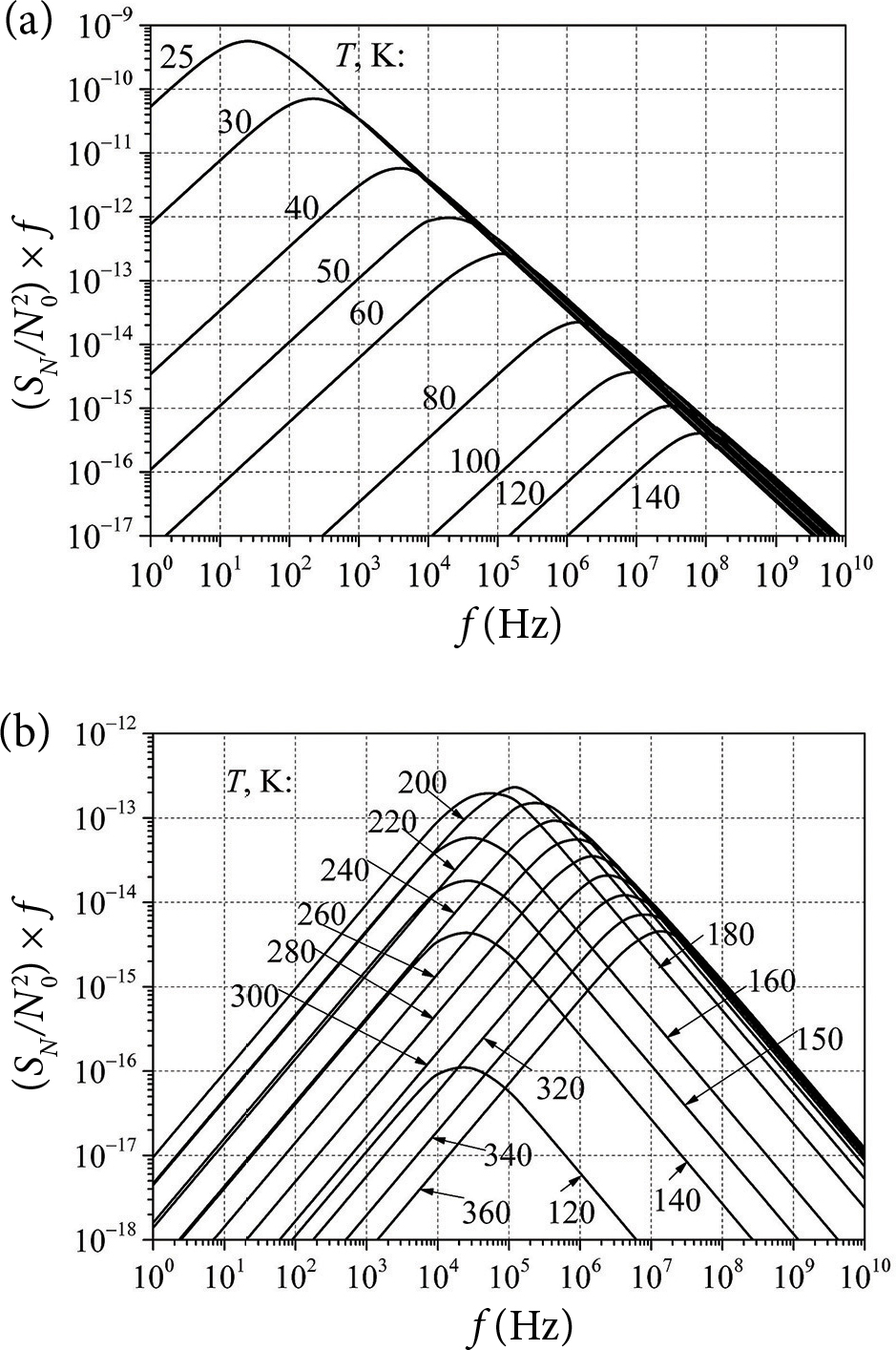Fig. 14. The normalized spectra of free electron number fluctuations caused by (a) the shallow donor level E1d = 0.05 eV with donor density n1d = 2·1013 cm–3 and (b) the donor level E2d = 0.25 eV with the donor density n2d = 3·1014 cm–3 at various temperatures for the silicon sample without the compensation by acceptors.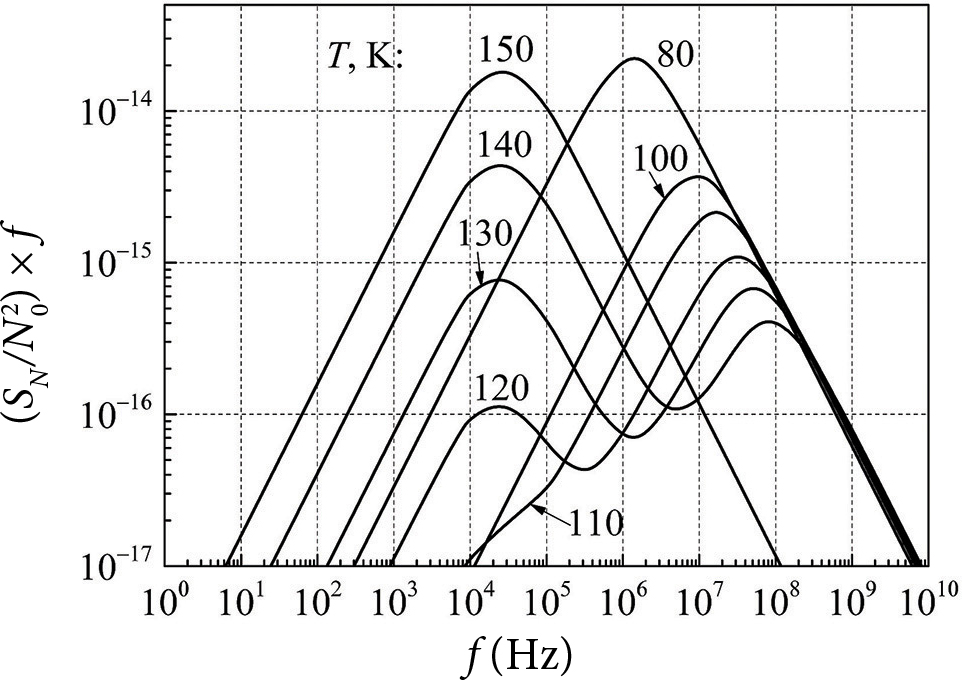Fig. 15. The normalized spectra of free electron number fluctuations for the acceptor free silicon sample with two donor levels (E1d = 0.05 and E2d = 0.25 eV) in the intermediate temperature range (100−150) K.

Consider the  noise spectra of the  same sample including the  compensation by acceptors. The spectral density of the g-r noise in this case can be described as

$\begin{array}{l}\frac{{S}_{N}\left(f\right)}{{N}_{0}^{2}}=4\frac{〈\Delta {n}_{1}^{2}〉}{{n}_{0}^{2}}\cdot \frac{{\tau }_{1}}{1+{\left(\omega {\tau }_{1}\right)}^{2}}\hfill \\ +4\frac{〈\Delta {n}_{2}^{2}〉}{{n}_{0}^{2}}\cdot \frac{{\tau }_{2}}{1+{\left(\omega {\tau }_{2}\right)}^{2}}.\hfill \end{array}\text{\hspace{0.17em}}\text{\hspace{0.17em}}\text{\hspace{0.17em}}\text{\hspace{0.17em}}\text{\hspace{0.17em}}\text{\hspace{0.17em}}\text{\hspace{0.17em}}\text{\hspace{0.17em}}\text{\hspace{0.17em}}\text{\hspace{0.17em}}\text{\hspace{0.17em}}\text{\hspace{0.17em}}\text{\hspace{0.17em}}\text{\hspace{0.17em}}\text{\hspace{0.17em}}\text{\hspace{0.17em}}\text{\hspace{0.17em}}\text{\hspace{0.17em}}\text{\hspace{0.17em}}\text{\hspace{0.17em}}\text{\hspace{0.17em}}\text{\hspace{0.17em}}\text{\hspace{0.17em}}\left(28\right)$

The calculation results obtained by Eq. (28) are presented in Fig. 16. The  dashed curves are due to the  free electron number fluctuations from the  shallow donor level E1d. The  dashed arrows show the  changes of the  g-r noise spectra when temperature increases. The solid curves represent the noise spectra caused by the free electron number fluctuations from the  deep donor level E2d. The  g-r noise spectra due to the  deep level donors always exceed the g-r noise level caused by the shallow donors at temperatures T > 100 K.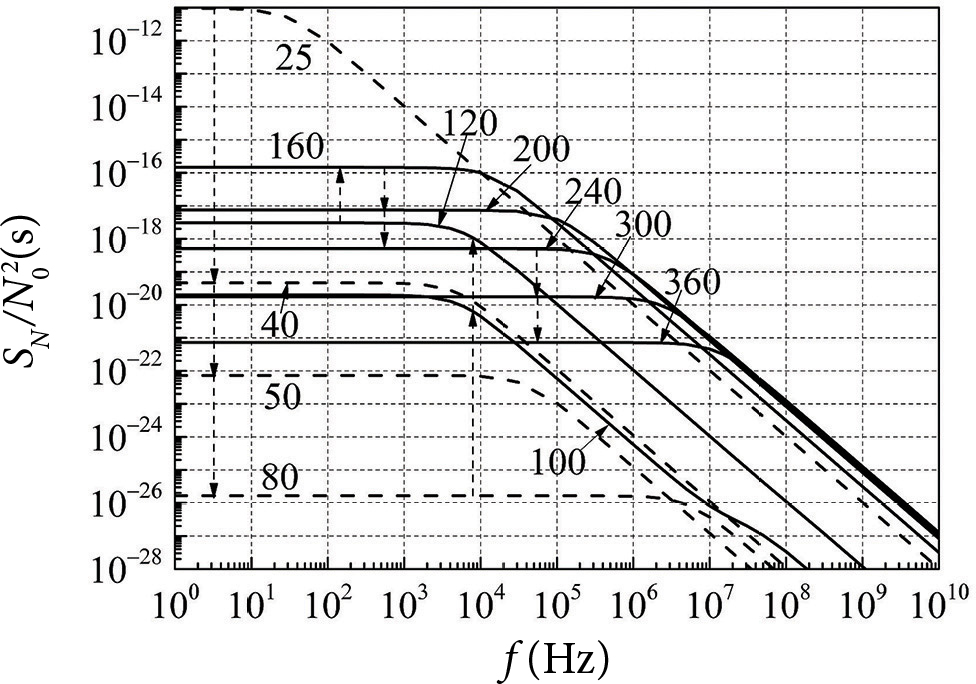Fig. 16. The relative g-r noise spectra SN/N02 for the partially compensated by acceptors (na = 1.5·1013 cm–3) silicon sample with the  shallow donor density n1d  =  2·1013  cm–3 at the  energy level E1d  =  0.05  eV and with the deep donor density n2d = 3·1014 cm−3 at the energy level E2d = 0.25 eV at various temperatures.

The results of the normalized g-r noise spectra calculated by Eq. (27) are presented in Fig. 17.

The comparison of the  calculation results for silicon samples with the same donor densities, but in one case without the compensation by acceptors (Fig. 14) and in another with partial compensation by acceptors, shows that the noise spectra presented in Fig. 17(a) and caused by the shallow donors have noticeable changes due to the influence of acceptors and free electrons from the deep donor level at temperatures T > 80 K. The noise spectra caused by the  deep donors (Fig. 17(b)) have almost the same pattern as in Fig. 14(b).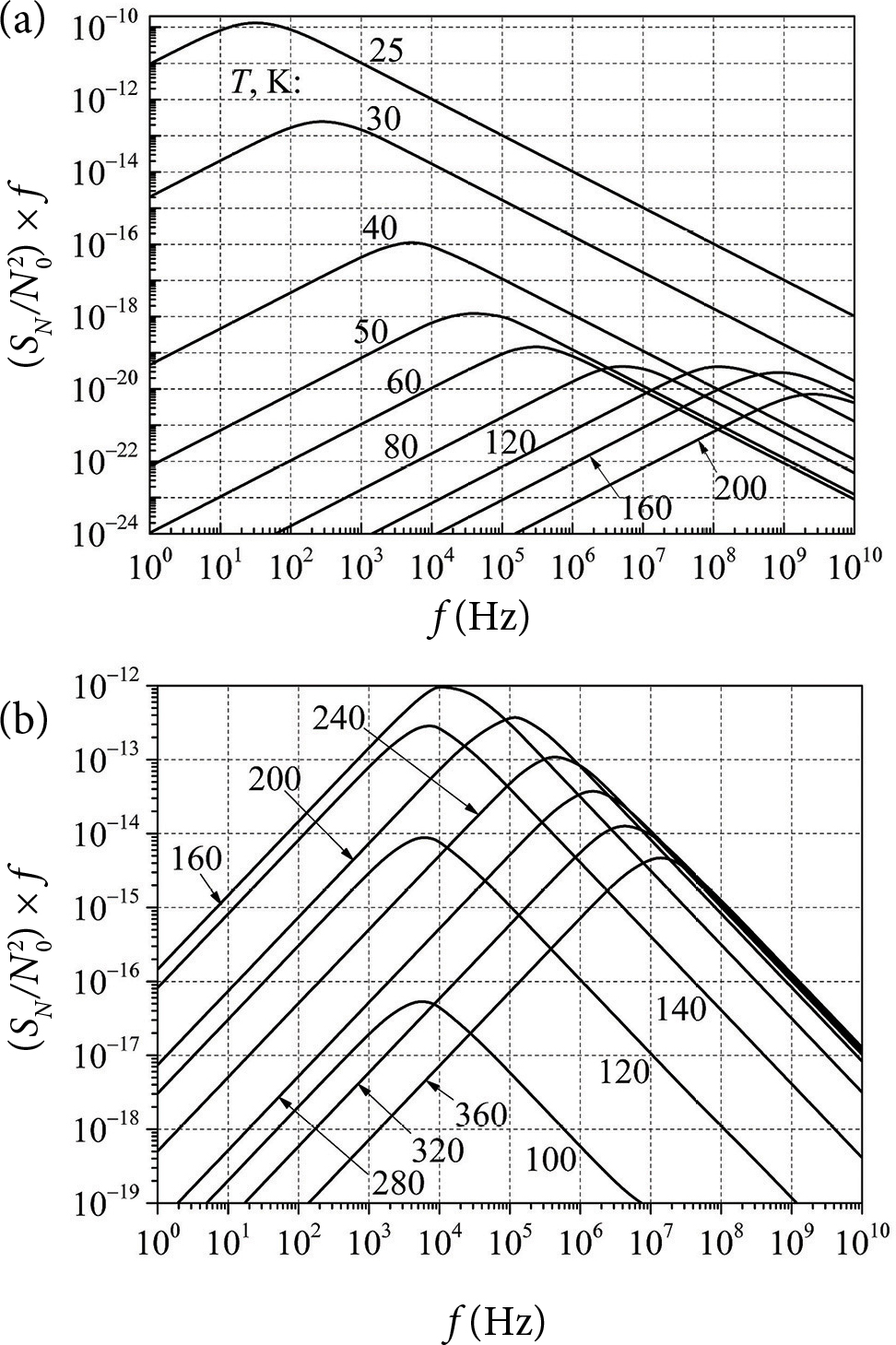Fig. 17. The normalized g-r noise spectra caused by (a) the shallow donor level E1d = 0.05 eV with the donor density n1d = 2·1013 cm–3, and (b) the deep donor level E2d = 0.25 eV with the donor density n2d = 3·1014 cm−3 in the partially compensated by acceptors (na = 1.5·1013 cm–3) silicon sample.

## 3. Conclusions

The generation-recombination processes in silicon have been analyzed in the temperature range from 20 to 360 K. It is demonstrated that the electron density strongly depends on temperature: the  shallow donors with a  density smaller than 1013 cm–3 are completely ionized at liquid nitrogen and higher temperatures, while only 20% of donors are ionized when the  density of shallow donors is about 1017 cm–3 at liquid nitrogen temperature. The  electrons fill the  energy states near the  bottom of the conduction band when donor density is low, but for the higher donor densities, some electrons must be excited to the higher energy levels of the conduction band, because the energy levels near the  bottom of the  conduction band are occupied, and the higher temperature is needed for excitation. It is also shown that in the transient region to the degenerate state, the standard Eq. (1) does not fully accurately describe the free electron density dependence on the shallow donor density. The revised formula (Eq. (13)), which is valid at any shallow donor density, is presented.

The maximum of the variance of free electron density fluctuations for a silicon sample with shallow donors strongly increases (about four orders of magnitude) with increasing donor density and shifts in the temperature range from 50 to 125 K. The  relative variance of free electron number fluctuations (〈∆N2〉/N0) is always equal to 0.5 at low temperatures and reflects the  situation when the free electron fluctuations obey the Poisson’s law.

The normalized spectra of the  generation-recombination noise in silicon with shallow and deep donor levels are presented in the frequency interval from 1 to 1010 Hz and the temperature range from 25 to 360 K. The comparison between the g-r noise spectra in silicon with and without the partial compensation by acceptors is also presented.

## References

 J. Machlup, Noise in semiconductor: spectrum of two parameter random signal, J. Appl. Phys. 25, 341−343 (1954).

 A. van der Ziel, Fluctuation Phenomena in Semiconductors (Butterworths Scientific Publications, London, 1969).

 J.A. Copeland, Semiconductor impurity analysis from low-frequency noise spectra, IEEE Trans. Electron. Dev. 18(1), 50−53 (1971).

 G.  Bosman and R.J.J.  Zijlstra, Generation-recombination noise in p-type silicon, Solid State Electron. 25(4), 273−280 (1982).

 F.  Hofman, R.J.J.  Zijlstra, J.M.B.  de  Freitas, and J.C.M. Hen ning, Generation-recombination noise in AlxGa1–xAs, Solid State Electron. 34(1), 23−32 (1991).

 Z. Šoblickas and V. Palenskis, Noise spectroscopy of impurity levels and 1/f noise in high-resistance silicon, Lith. J. Phys. 25, 88−97 (1985).

 L. Varani, L. Reggiani, V. Mitin, K.M. Van Vliet, and T. Kuhn, Nonexponential generation-recombination dynamics in doped semiconductors as a  possible source of high-frequency 1/f noise, Phys. Rev. B 48, 4405−4411 (1993).

 A. van der Ziel, Noise: Sources, Characterization, Measurement (Prentice-Hall, Inc., Englewood Cliffs, New York, 1970).

 A. van der Ziel, Noise in Solid State Devices and Circuits (John Wiley & Sons, Inc., New York, 1986).

 C.F. Hiat, A. van der Ziel, and K.M. Van Vliet, Generation-recombination noise produced in the channel of JFET’s, IEEE Trans. Electron. Dev. 22(8), 614−616 (1975).

 J.  Matukas and V.  Palenskis, Generation-recombination noise in silicon p+-n-n+ junction with strongly compensated n-region, Fizika i Tekhnika Poluprovodnikov 18, 1721−1723 (1984) [in Russian].

 V.  Mitin, L.  Reggiani, and L.  Varani, in: Noise and Fluctuations Control in Electronic Devices, ed. A.A.  Balandin (American Scientific Publishers, California, 2002).

 V.  Palenskis, J.  Matukas, S.  Pralgauskaitė, J.G. Sim mons, S. Smetona, and R. Sobiestianskas, Experi mental investigations of the  effect of the  mode-hopping on the  noise properties of InGaAsP Fabry-Pérot multiple-quantum well laser diodes, IEEE Trans. Electron. Dev. 50(2), 366–371 (2003).

 V. Palenskis, J. Matukas, J. Vyšniauskas, S. Pralgaus kaitė, H. Shtrikman, D. Seliuta, I. Kašalynas, and G. Valušis, Analysis of noise characteristics of GaAs tunnel diodes, Fluct. Noise Lett. 12(3), 1350014 (2013).

 J. Glemža, V. Palenskis, S. Pralgauskaitė, J. Vyšniaus kas, and J. Matukas, Properties of the surface generation-recombination noise in 1.94 µm GaSb-based laser diodes, Infrared Phys. Technol. 91, 101–106 (2018).

 M.J. Kirton and M.J. Uren, Noise in solid-state microstructures: A new perspective on individual defects, interface states and low-frequency (1/f) noise, Adv. Phys. 38(4), 367–468 (1989).

 V. Palenskis, K. Maknys, A. Stadalnikas, Z. Šoblic kas, and A.  Utorovičius, in: Proceedings of the  7th International Conference on Fluctuation Phenomena in Physical Systems, ed. V. Palenskis (VU Press, Vilnius, 1994).

 V. Palenskis, The charge carrier capture-emission process – the main source of the low-frequency noise in homogeneous semiconductors, Lith. J. Phys. 56(4), 200–206 (2016).

 V. Palenskis, J. Vyšniauskas, J. Glemža, and J. Matukas, Charge carrier mobility fluctuations due to the capture-emission process, Lith. J. Phys. 58(3), 261–266 (2018).

 J.S.  Blakemore, Semiconductor Statistics (Pergamon Press, Oxford, New York, 1962).

 T.N. Morgan, Broadening of impurity bands in heavily doped semiconductors, Phys. Rev. 139, A343 (1965).

 G.L. Pearson and J. Bardeen, Electrical properties of pure silicon and silicon alloys containing boron and phosphorus, Phys. Rev. 75(5), 865–883 (1949).

 N.  Kobayashi, S.  Ikehata, S.  Kobayashi, and W. Sa saki, Specific heat study of heavily P doped Si, Solid State Commun. 24(1), 67–70 (1977).

 F.J. Morin and J.P. Maita, Electrical properties of silicon containing arsenic and boron, Phys. Rev. 96(1), 28–35 (1954).

### V. Palenskis et al. / Lith. J. Phys. 62, 148–160 (2022)

#### GENERACINIS-REKOMBINACINIS TRIUKŠMAS IR KITOS LEGIRUOTO SILICIO YPATYBĖS PLAČIAME TEMPERATŪROS INTERVALE

V. Palenskis, J. Glemža, J. Matukas

Vilniaus universiteto Taikomosios elektrodinamikos ir telekomunikacijų institutas, Vilnius, Lietuva

## Santrauka

Silicio generacinio-rekombinacinio triukšmo charakteristikos yra ištirtos temperatūros intervale nuo 25 iki 360  K. Ypač didelis dėmesys skirtas siliciui su sekliomis donorinėmis priemaišomis, esant labai plačiam donorų tankiui. Parodyta, kad laisvųjų elektronų tankis stipriai priklauso nuo temperatūros net sekliųjų donorų (donorinio lygmens energija lygi 0,05 eV) atveju. Seklieji donorai, esant jų tankiui mažesniam nei 1013 cm–3, skysto azoto temperatūroje yra visiškai jonizuoti, o sekliųjų donorų, kurių tankis yra apie 1017 cm–3, tik apie 20 % yra jonizuoti. Esant mažam sekliųjų donorų tankiui, laisvieji elektronai užpildo energijos lygmenis, esančius arti laidumo juostos dugno. Didėjant donorų tankiui, dalis elektronų turi būti sužadinami į aukštesnius laidumo juostos energijos lygmenis, todėl jiems sužadinti reikalinga aukštesnė temperatūra. Taip pat parodyta, kad pereinamojoje link išsigimusių elektroninių dujų srityje standartinis sąryšis (1) ne visai tiksliai parodo laidumo juostos elektronų tankio priklausomybę nuo sekliųjų donorų tankio. Pateikta patikslinta išraiška (13), kuri galioja esant bet kokiam sekliųjų donorų tankiui.

Minėtos sekliųjų donorų ypatybės pasireiškia ir generacinio-rekombinacinio triukšmo charakteristikose. Generacinio-rekombinacinio triukšmo dispersijos maksimumas dėl laisvųjų elektronų tankio fliuktuacijų silicyje, kylant sekliųjų donorų tankiui nuo 1013 iki 1017 cm–3, stipriai didėja (apie 4 eiles), ir šis maksimumas pasislenka temperatūros skalėje nuo 50 iki 125 K. Parodyta, kad laisvųjų elektronų skaičiaus N fliuktuacijų dispersija (〈∆N2〉/N0) žemoje temperatūroje visada lygi 0,5, ir tai rodo, kad žemoje temperatūroje laisvųjų elektronų skaičiaus fliuktuacijos apibūdinamos Puasono (Poisson) tikimybių pasiskirstymo dėsniu.

Normuoto generacinio-rekombinacinio triukšmo spekt rų [SN(f)/N 02 × f] dažninės priklausomybės yra pateiktos in ter vale nuo 1 iki 1010 Hz temperatūros srityje nuo 25 iki 360 K. Taip pat pateiktos silicio generacinio-rekombinacinio triukšmo charakteristikos, esant dviem donoriniams lygmenims, įskaitant kompensavimą akceptoriais.